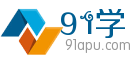面向未来，活在当下！ 收藏夹

 当前：首页 > 数学 > 高中题型 > 高考数学必做百题(理)
 高考数学必做百题第01题（理科2017版）001.（1）（2016新课标Ⅰ理2）设集合 $A=\{x|{{x}^{2}}-4x+3<0\}$ ，$B=\{x|2x-3>0\}$，则$A\bigcap B=$（ ） A.$\left( -3,-\frac{3}{2} \right)$ B.$\left( -3,\frac{3}{2} \right)$【答案详解】
 高考数学必做百题第02题（理科2017版）002. 已知集合$A=\{x|3\le x<7\}$，$B=\{x|5  高考数学必做百题第03题（理科2017版） 003. 已知集合$A=\left\{ {{a}^{2}},a+1,-3 \right\}$，$B=\left\{ a-3,a-2,{{a}^{2}}+1 \right\}$。（1）若$A\bigcap B=\left\{ -3 \right\}$，求A∪B；（2）存在实数$a$，使得$A=B$吗【答案详解】  高考数学必做百题第04题（理科2017版） 004. 设集合$A=\{x|x\left( {{x}^{2}}-x-2 \right)=0\}$，$B=\{x|a{{x}^{2}}=1\}$. （1）若$B\subseteq A$，求实数a的值；（2）若$a=4$，求$A\bigcup B$的真子集个数。解：（1）$A=\left\{ \tex【答案详解】
 高考数学必做百题第05题（理科2017版） 005.（1）已知p：x2 －8x－20≤0，q：x2 －2x＋1－a2 ≤0(a>0)．若p是q的充分不必要条件，则实数$a$的取值范围是________。（2）已知命题p：方程x2 +mx+1=0有两个不相等的负实根，命题q：不等式4x2 +4(m-【答案详解】
 高考数学必做百题第06题（理科2017版） 006.（1）（2016山东理11）执行右边的程序框图，若输入的a,b的值分别为0和9，则输出的i的值为________。（2）求$\frac{1}{{{2}^{2}}-1}+\frac{1}{{{4}^{2}}-1}+\frac{1}{{{6}^{2}}-1}+\cdo【答案详解】  高考数学必做百题第07题（理科2017版） 007. （1）已知平面向量$\overrightarrow{a}=\left( 1,x \right),\ \overrightarrow{b}=\left( 2x+3,-x \right)$(x∈R)，若$\overrightarrow{a}//\overrightarrow{b}$，则$\l【答案详解】
 高考数学必做百题第08题（理科2017版） 008.已知点$G$是$\Delta ABO$的重心，$M$是$AB$边的中点。（1）求$\overrightarrow{GA}+\overrightarrow{GB}+\overrightarrow{GO}$；（2）若$PQ$过$\Delta ABO$的重心$G$，且$\overright【答案详解】  高考数学必做百题第09题（理科2017版） 009.已知$\left| \overrightarrow{a} \right|=4,\left| \overrightarrow{b} \right|=3$，$(2\overrightarrow{a}-3\overrightarrow{b})\cdot (2\overrightarrow{a}+\overrigh【答案详解】
 高考数学必做百题第10题（理科2017版） 数系的扩充与复数的引入010.（1）（2016天津理9）已知$a,b\in R$，i是虚数单位，若$(1+i)(1-bi)=a$，则$\frac{a}{b}$的值为_______。（2）已知复数${{z}_{1}}$满足$\left( {{z}_{1}}-2 \righ【答案详解】  高考数学必做百题第11题（理科2017版） 011.（1）设i是虚数单位，$\overline{z}$是复数$z$的共轭复数，若$z\cdot \overline{z}i+2=2z$，则$z$＝( )$A$．$1+iB$．$1-iC$．$-1+iD$．$-1-i$（2）$\frac{{{\lef【答案详解】
 高考数学必做百题第12题（理科2017版） 函数012. （1）（2016天津13）已知$f\left( x \right)$是定义在$R$上的偶函数，且在区间$\left( -\infty .0 \right)$上单调递增.若实数$a$满足$f({{2}^{\left| a-1 \right|}})>f(-\s【答案详解】  高考数学必做百题第13题（理科2017版） 013. 已知函数$f(x)=\frac{ax+1}{2-x}\left( a\ne 0 \right)$。（1）求$f(x)$的定义域与值域； （2）讨论$f(x)$在$(2,+\infty )$上单调性。解：（1）要使函数有意义，则$2-x\ne 0$，解得$x\ne【答案详解】
 高考数学必做百题第14题（理科2017版） 014.设$f\left( x \right)=\frac{{{e}^{-x}}}{a}+\frac{a}{{{e}^{-x}}}$是定义在$R$上的函数。（1）$f\left( x \right)$可能是奇函数吗？（2）若$f\left( x \right)$是偶函数，求$a$的【答案详解】
 高考数学必做百题第15题（理科2017版） 015. 已知函数f(x)=\left\{ \begin{align} & x(x+3),x\ge 0 \\ & x(x-3),x<0 \\ \end{align} \right.，（1）求$f\left( -1 \right)$,$f\left( 2 \right)$,$f(a+2)$的值；（2）判断$【答案详解】  高考数学必做百题第16题（理科2017版） 016. 已知函数$f(x)={{\log }_{a}}(x+3)$，$g(x)={{\log }_{a}}(3-x)$，其中$(a>0\ a\ne 1\ )$。 （1）求函数$f(x)+g(x)$的定义域； （2）判断$f(x)+g(x)$的奇偶性，并说明理由；（3）求使$f(x)【答案详解】
 高考数学必做百题第17题（理科2017版） 017.若函数$f(x)={{a}^{x}}(a>0,a\ne 1)$在[-1,2]上的最大值为4，最小值为$m$，且函数$g(x)=(1-4m)\sqrt{x}$在$[0,+\infty )$上是增函数，求实数$a$与$m$的值。解：当$a>1$时，则${{【答案详解】  高考数学必做百题第18题（理科2017版） 018.已知函数$f\left( x \right)={{\log }_{\frac{1}{2}}}\frac{ax-2}{x-1}$($a$为常数)．（1）若常数$a<2$且$a\ne 0$，求$f\left( x \right)$的定义域；（2）若$f\left( x \right)$在【答案详解】  高考数学必做百题第19题（理科2017版） 019.（1）已知函数$f\left( x \right)$有三个零点，且对一切实数$x$都满足$f\left( 1+x \right)=f\left( 1-x \right)$，求三个零点的和。（2）已知二次函数$f\left( x \right)=2{{x}^{【答案详解】
 高考数学必做百题第20题（理科2017版） 020.已知函数$f(x)={{x}^{2}}+2mx+2,x\in [-5,5]$（1）当$m=-2$时，求$f(x)$的最大值和最小值；（2）求实数$m$的取值范围，使$y=f(x)$在区间$[-5,5]$上是单调函数；（3）在（1）的条件下，设$g(x)=f(【答案详解】  高考数学必做百题第21题（理科2017版） 021.已知函数$f\left( x \right)={{x}^{2}}+bx+c\left( x\in R \right)$，且满足$f\left( 1 \right)=0$。（1）若函数$f\left( x \right)$有两个不同的零点，求$b$的取值范围；（2）若对$【答案详解】
 高考数学必做百题第22题（理科2017版） 022.如图，等腰梯形$OABC$的底角为${{60}^{\circ }}$，$OA=2$，$BC=1$，记梯形$OABC$位于直线$x=t(t>0)$左侧的图形的面积为$f(t)$. 试求函数$f(t)$的解析式，并画出函数$y=f(t)$的大【答案详解】
 高考数学必做百题第23题（理科2017版） 023.已知一扇形的圆心角为$\alpha \)$\left( \alpha >0 \right)\)，所在圆的半径为$R\)。（1）若$\alpha ={{60}^{\circ }}\)，$R=10cm\)，求扇形的弧长及该弧所在的弓形的面积；（2）若扇【答案详解】  高考数学必做百题第24题（理科2017版）024.已知角$\alpha $的终边经过$P\left( m,-3 \right)$，且$\cos \alpha =\dfrac{m}{5}$。（1）判断角$\alpha $的象限，求$3\sin \alpha -4\tan \alpha $的值； （2）化简$\dfrac{\sin【答案详解】
 高考数学必做百题第25题（理科2017版）025.（1）（2016四川理3）为了得到函数$y=\sin \left( 2x-\dfrac{\text{ }\!\!\pi\!\!\text{ }}{3} \right)$的图象，只需把函数$y=\sin 2x$的图象上所有的点（ ）A．向左平行移动\$\dfrac【答案详解】

（微信中可直接长按微信打赏二维码。）
 微信 支付宝